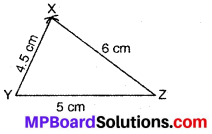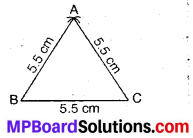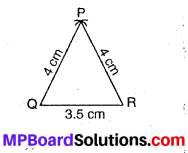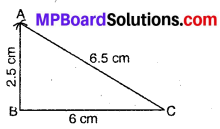## MP Board Class 7th Maths Solutions Chapter 10 Practical Geometry Ex 10.2

Question 1.
Construct ∆XYZ in which XY = 4.5 cm, YZ = 5 cm and ZX = 6 cm.
Solution:Steps of Construction :
1. Draw a line segment YZ = 5 cm.
2. With centre Y and radius 4.5 cm, draw Y an arc.
3. With centre Z and radius 6 cm draw another arc intersecting the previous arc at X.
4. Join YX and ZX. Then, XYZ is the required triangle.

Question 2.
Construct an equilateral triangle of side 5.5 cm.
Solution:
Steps of Construction :1. Draw a line segment BC = 5.5 cm.
2. With B as centre and radius equal to 5.5 cm (= BA), draw an arc.
3. With C as centre and radius equal to 5.5 cm (= CA), draw another arc intersecting the previous arc at A.
4. Join AB and AC.
Then, ABC is the required equilateral triangle.Question 3.
Draw ∆PQR with PQ = 4 cm, QR = 3.5 What type of triangle is this?
Solution:
Steps of Construction :1. Draw a line segment QR = 3.5 cm.
2. With Q as centre and radius equal to 4.0 cm (= QP), draw another arc intersecting the previous arc at P.
3. With R as centre and radius equal to 4.0 cm (= PR), draw another arc intersecting the previous arc at P.
4. Join PQ and PR
Then, PQR is the required triangle.
From above construction, ∆PQR is an isosceles triangle.

Question 4.
Construct ∆ABC such that AB = 2.5 cm, BC = 6 cm and AC = 6.5 cm. Measure ∠B.
Solution:
Steps of Construction :1. Draw a line segment BC = 6 cm.
2. With B as centre and radius equal to 2.5 cm. (AB) draw an arc.
3. With C as centre and radius equal to 6.5 cm (AC), draw another arc intersecting the
4. Join AB and AC.
Then, ABC is the required triangle.
On measuring, we find that ∠B = 90°.